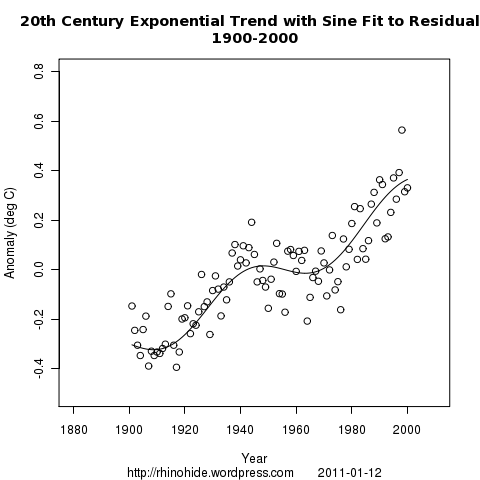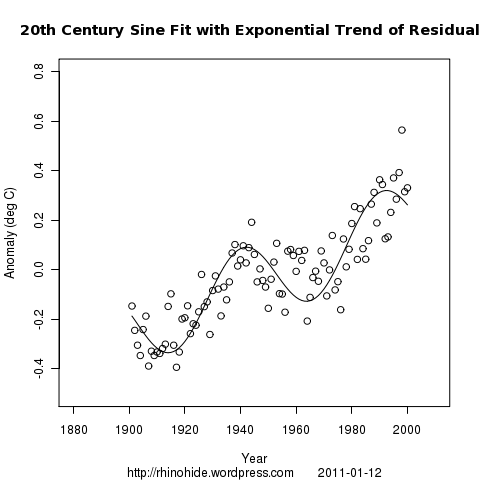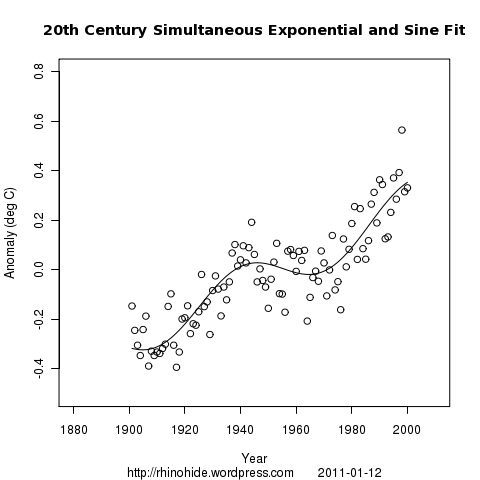Home > GIStemp, LSCF > Lines, Sines, and Curve Fitting 5 – a growth

Lines, Sines, and Curve Fitting 5 – a growth

2011 January 12

From the comments in Curve Fitting 4:

If you want to try a different flavor of gum, consider simultaneously fitting exponential + sine models:

y1(t) = y1(0) * exp(kt)
y2(t) = A * sin(((t-b)/T) * 2 * pi)
y(t) = y1(t) + y2(t)

You’ve probably already thought of this, of course.

Ned’s been peeking ahead, so maybe I shouldn’t “reward” him. 😉 But here it is anyway. The procedures are similar. I fitted an exponential with a sine of the residuals, a sine with an exponential of the residuals, and fitting both a sine and exponential simultaneously. I know that there are pre-packaged R methods for fitting exponentials, but I use the method of looking for best fit in by looping through the parameter space.

The form of the exponential that I used was: y1= -a/100 + (bb/10) * exp((k/10000)*(YEARS-1880))Exp a = 149
Exp bb = 11
Exp k = 40

Sine Amplitude A = 8
Sine Phase Shift b = 24
Sine Period T = 56Sine Amplitude A = 16
Sine Phase Shift b = 24
Sine Period T = 50

Exp a = 130
Exp bb = 10
Exp k = 34Exp a = 145
Exp bb = 11
Exp k = 37

Sine Amplitude A = 9
Sine Phase Shift b = 34
Sine Period T = 61

1. 2011 January 12 at 11:36 am

That was quick. It looks similar to my version. Thank you!

I am curious about where you are leading us with all of this curve-fitting. Are you just doing “exploratory data analysis” here, or are you building up to something? No need to actually answer that if you don’t want to; I will wait and see.

2. 2011 January 12 at 11:47 am

I figured that before I went snipe-hunting, I should do some target practise first.

Part of it is just to get better at R. Another is to get more familiar with equations which are statistically justifiable. A third is to walk slowly towards better tools: Fourier, Arima, ACF, PCA. Confidence intervals in complex eqns. Auto-correlation. There is lots of things right for me to learn some basics and not-so-basics.

But, no, I don’t have a pre-planned conclusion in mind. This is stream-of-consciousness, baby-steps in the dark stuff. But I would like to be able to better analyze the claims of 1500,1100,800,180,60,22,11 or whatever cycles. So there will be some stuff along those lines eventually.

1. 2011 February 13 at 10:41 am
2. 2011 March 10 at 8:27 pm